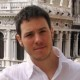# Geometric Rhythm Machine

In the post Playing with laser beams to create very simple rhythms” I explained a theoretical approach that I want to materialize into an instrument. The idea is to create complex rhythms by combining several times the same rhythmic patterns, but each time with some variation compared to the original pattern.

Several possible variations (or transforms, since a variation is generated by applying a transform to the original pattern) were proposed, starting from an hypothetical rhythmic pattern “x.x.xx..”. Three linear transforms: Reverse (”..xx.x.x”), Roll (”x.x.xx..”) and Scale 2:1 (”x…x…x.x…..”) or 1:2 (”xxx.”), and some non-linear transforms: Truncate (”xx..”) etc.

# Geometry + Light = Tangible transforms

The very idea behind the various experiments made using laser light beams and LDR sensors is to build an instrument that proposes all the previous transforms in a tangible fashion: when you move physical object, you also change the rhythm accordingly.

Let’s consider a very narrow light beam turning just like the hands of a clock. Let’s suppose that our clock has no number written around, but we can position marks (mirrors) wherever on the clock surface. Still in the context of creating rhythms, now assume that every time a hand crosses a mark (mirror) we trigger a sound. So far we have a rhythmic clock, which is a funny instrument already. But we can do better…

Going back to our rhythmic pattern “x.x.xx..”, we can represent it with 4 mirrors that we position on a same circle. On the illustration below this original pattern is displayed in orange, each mirror shown by an X letter.. If we now link these 4 mirrors together with some adhesive tape, we have built a physical object that represents a rhythmic pattern. The rotating red line represents the laser beam turning like the clock hands.Illustration of how the geometry of the rhythmic clock physically represents the transforms

Now we have a physical pattern (the original one), we can of course create copies of it (need more mirrors and more adhesive tape). We can then position the copies elsewhere on the clock. The point is that where you put a copy defines the transform that applies to it compared with the original pattern:

• If we just shift a pattern left or right while remaining on the same circle, then we are actually doing the Roll transform (change the phase of the pattern) (example in blue on the picture)
• If we reverse the adhesive tape with its mirrors glued on, then of course we also apply the Reverse transform to the pattern (example in grey on the picture)
• If we move the pattern to another (concentric) circle, then we are actually applying the Scale transform, where the scaling coefficient is the fraction of the radius of the circle over the radius of the circle of the original pattern (example in green on the picture)

Therefore, simple polar geometry is enough to provide a complete set of physical operations that fully mimic the desired operations on rhythmic pattern. And since this geometry is in deep relationship with how the rhythm is being made, the musician can understand what’s happening and how to place the mirrors to get any the desired result. The system is controllable.

To apply the Truncate transform (that is not a linear transform) we can just stick a piece of black paper to hide the mirror(s) we want to mute.

If we layer the clock we just described, with one layer for each timbre to play, then again changing the timbre (yet another non-linear transform) can then be done by physically moving the pattern (mirrors on adhesive tape) across the layers.

# From theory to practice

Though appealing in principle, this geometric approach is hard to implement into a physical installation, mostly because accuracy issues:

1. The rotating mirror must rotate perfectly, with no axis deviation; any angular deviation is multiplied by two and then leads to important position deviation in the light spot in the distance: delta = distance . tan(2 deviation angle)
2. Each laser module is already not very accurate: the light beam is not always perfectly aligned with the body. To fix that would require careful tilt and pan adjustment on the laser module support
3. Positioning the retroreflectors in a way that is accurate and easy to add, move or remove at the same time is not that easy; furthermore, even if in theory the retroreflectors reflect all the incoming light back to where it comes from, in practice maximum reflectance happens when the light hits the reflector orthogonally, which is useful to prevent missed hits

Don’t hesitate to check these pages for progress, and any feedback much appreciated.#### cyrille

Software development, Domain-Driven Design, patterns and agile principles enthusiast

## 2 thoughts on “Geometric Rhythm Machine”

1. Pingback: trends watch